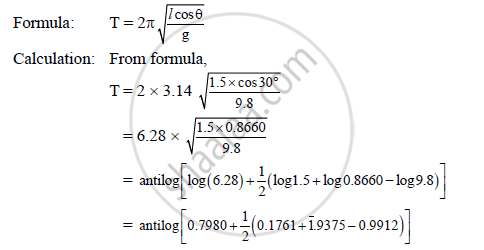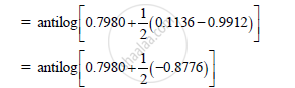# A Stone of Mass 2 Kg is Whirled in a Horizontal Circle Attached at the End of 1.5m Long String. If the String Makes an Angle of 30° with Vertical, Compute Its Period - Physics

A stone of mass 2 kg is whirled in a horizontal circle attached at the end of 1.5m long string. If the string makes an angle of 30° with vertical, compute its period. (g = 9.8 m/s2)

#### Solution

Given: m = 2 kg

l = 1.5 m

Θ = 30°

g = 9.8 m/s2

To find: Period (T)= antilog[0.3592]

∴ T = 2.2875 s

Period of revolution is 2.287 s

Concept: Rolling Motion
Is there an error in this question or solution?
2015-2016 (July)

Share